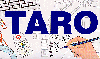The Traffic Accident Reconstruction Origin -Article-[Home] [ARnews] [Contents] [Classified] [Advertisers] [Approach Angles] [E-mail Directory] [Feedback] [Organizations] [Reference Library]

Error Analysis for Drag Sleds

Method 2- A Drag Sled

Drag sled convention recommends multiple pulls across the surface in question. Once ten readings are taken. Those results are averaged to find the value of drag factor. The following data was obtained in a drag sled test on a paved surface.

Time: 9:50 AM, Date 6/6/86, sled weight: 54 pound sled, Scale: Hanson Model 8920, Number of Samples: 10 (Drag sled test conducted by J.F. (Jim) Mitchell jfmitch@bconnex.net)

Pull Data (pounds)

 42 40 40 38 38 40 42 38 40 41

We will use the following Equation to determine uncertainty: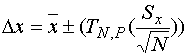Equation 1

Where,= 39.9 - The average (mean) of the tests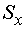= 1.52 - The Standard Deviation of the tests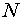= 10 - The Number of tests in the sample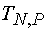= 2.26 - The t estimator from the table with 10 samples at 95% probability= 1.08 Our answer for uncertainty- The range in which the actual value of x lies at the given probability. Where:

We must also find the uncertainty in the value of weight of the drag sled. We will assume the scale to be calibrated. We will also assume that we are able to read to half the values incremented on the scales index. The scale is indexed every 2 pounds, so the resulting uncertainty is 1 pound.

Next, we must examine how these uncertainties propagate through the equation to drag factor.

The Equation for drag factor from a drag sled isUncertainty propagates to the measured value through the partial derivatives of this equation. Those partial derivatives are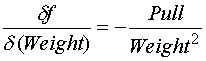Using the Average value for Pull calculated above as 39.9 lbs. and Weight of the sled as 54 lbs. yields the following sensitivities.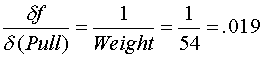Using the uncertainty value for Pull calculated above as 1.09, and an uncertainty of 1(half the scale's incremented value) for Weight yields the following for uncertainty propagated to the calculated drag factor.

Uncertainty in pull propagated to drag factor is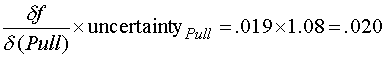Uncertainty in Weight propagated to drag factor is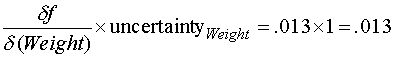And finally, the total uncertainty in drag factor is the sum of these two values or .02+.013=.033

So, we can express the results of this drag sled test as follows: There is a 95% probability that the true value of the drag factor is .74 +- .033.